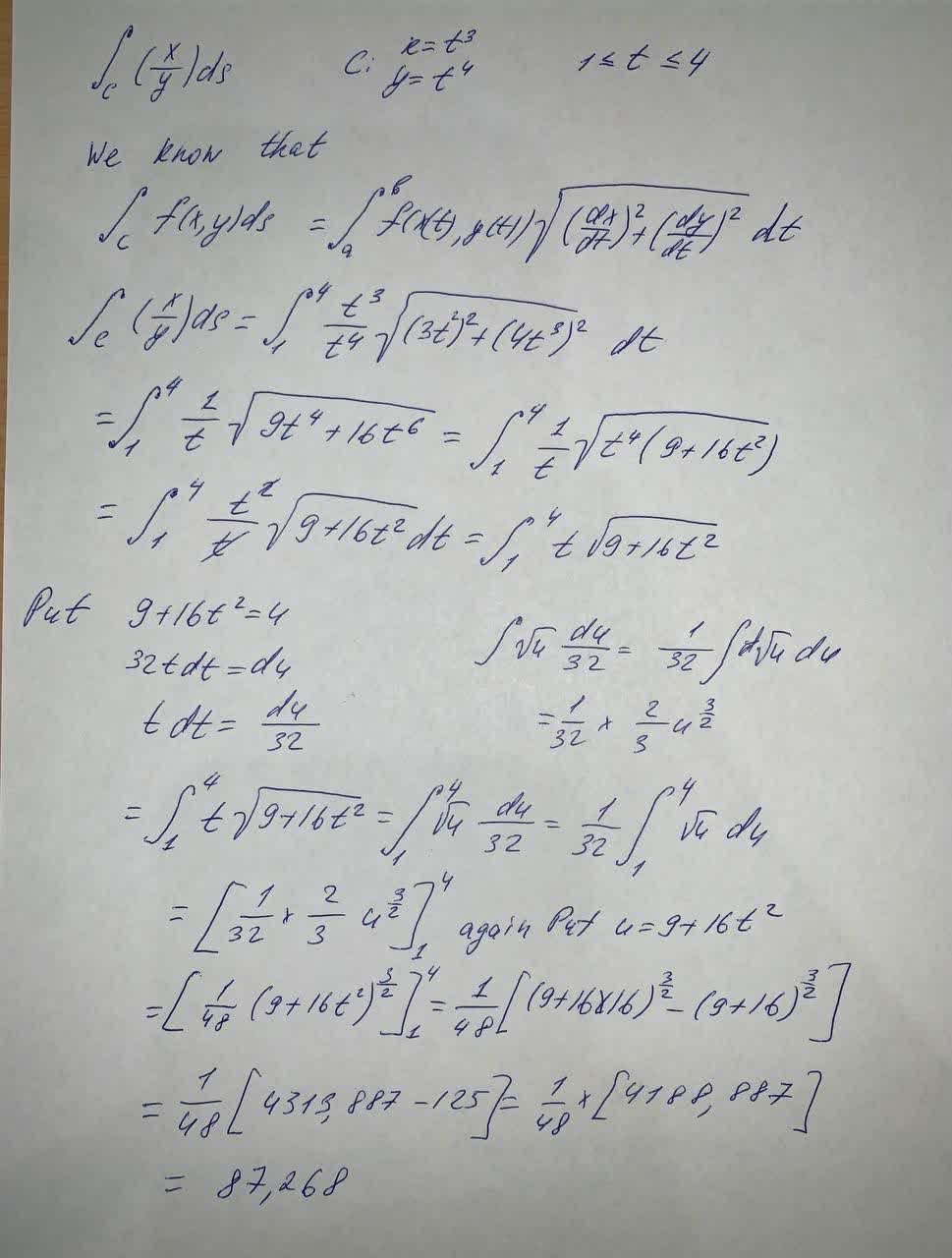Question# Evaluate the line integral, where C is the given curve \int_C (\frac{x}{y})ds , C:x=t^3 , y=t^4 , 1 \leq t \leq 4

Integrals
ANSWERED$$\int_C (\frac{x}{y})ds , C:x=t^3 , y=t^4 , 1 \leq t \leq 4$$